### 最终效果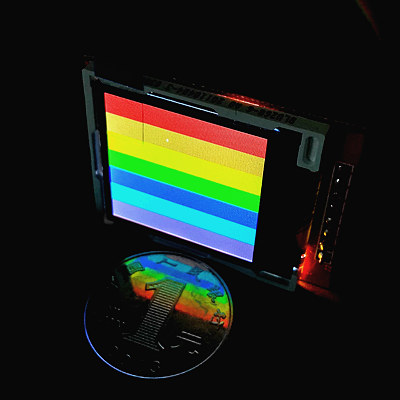### 硬件

• 1.44英寸 spi接口 128x128液晶屏幕 X 1（淘宝10-20元）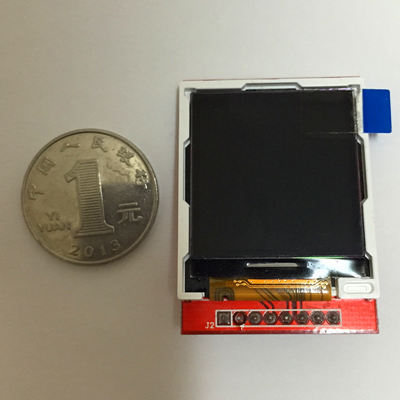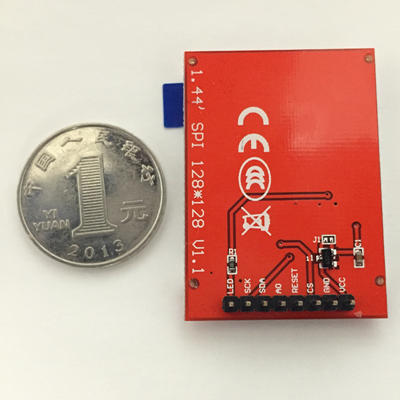### 先上代码看效果

#!/usr/bin/env python

import RPi.GPIO as GPIO
import time

cs=23        # 片选
rs=17        # 数据 / 命令 切换
sda=13        # 数据
scl=19        # 时钟
reset=27    # 复位

# 传输byte
def setByteData(data):
# print ""
# print "S-----------setByte---------------:", hex(data)
for bit in range(0,8):
# 传入的数字从高位到低位依次判断是否为1，若为1则设置高电平，否则设置低电平
# 判断的方法是先向左移位，把要判断的位移动到最高位然后跟0x80（1000 0000）相与，
# 如果结果仍然是0x80（1000 0000）就表示最高位是1，否则最高位就是0
if ((data<<bit) & 0x80 == 0x80):
setBitData(True)
# print "1",
else:
setBitData(False)
# print "0",
# print ""
# print "E-----------setByte---------------"

def setBitData(data):
GPIO.output(scl, False)
GPIO.output(sda, data)
GPIO.output(scl, True)

def write_command(cmd):
GPIO.output(cs, False)
GPIO.output(rs, False)
setByteData(cmd)
GPIO.output(cs, True)

def write_data(data):
GPIO.output(cs, False)
GPIO.output(rs, True)
setByteData(data)
GPIO.output(cs, True)

def write_data_16bit(dataH, dataL):
write_data(dataH)
write_data(dataL)

def lcd_reset():
GPIO.output(reset, False)
time.sleep(0.1)
GPIO.output(reset, True)
time.sleep(0.1)

def lcd_init():
lcd_reset()

write_command(0x11) # Exit Sleep
time.sleep(0.02)
write_command(0x26) # Set Default Gamma
write_data(0x04)
write_command(0xB1)# Set Frame Rate
write_data(0x0e)
write_data(0x10)
write_command(0xC0) # Set VRH1[4:0] & VC[2:0] for VCI1 & GVDD
write_data(0x08)
write_data(0x00)
write_command(0xC1) # Set BT[2:0] for AVDD & VCL & VGH & VGL
write_data(0x05)
write_command(0xC5) # Set VMH[6:0] & VML[6:0] for VOMH & VCOML
write_data(0x38)
write_data(0x40)

write_command(0x3a) # Set Color Format
write_data(0x05)
write_command(0x36) # RGB
write_data(0xc8)

write_data(0x00)
write_data(0x00)
write_data(0x00)
write_data(0x7F)
write_data(0x00)
write_data(0x00)
write_data(0x00)
write_data(0x7F)

write_command(0xB4)
write_data(0x00)

write_command(0xf2) # Enable Gamma bit
write_data(0x01)
write_command(0xE0)
write_data(0x3f)# p1
write_data(0x22)# p2
write_data(0x20)# p3
write_data(0x30)# p4
write_data(0x29)# p5
write_data(0x0c)# p6
write_data(0x4e)# p7
write_data(0xb7)# p8
write_data(0x3c)# p9
write_data(0x19)# p10
write_data(0x22)# p11
write_data(0x1e)# p12
write_data(0x02)# p13
write_data(0x01)# p14
write_data(0x00)# p15
write_command(0xE1)
write_data(0x00)# p1
write_data(0x1b)# p2
write_data(0x1f)# p3
write_data(0x0f)# p4
write_data(0x16)# p5
write_data(0x13)# p6
write_data(0x31)# p7
write_data(0x84)# p8
write_data(0x43)# p9
write_data(0x06)# p10
write_data(0x1d)# p11
write_data(0x21)# p12
write_data(0x3d)# p13
write_data(0x3e)# p14
write_data(0x3f)# p15

write_command(0x29) #  Display On
write_command(0x2C)

def show_single_color(DH,DL):
for i in xrange(0,128):
for j in xrange(0,128):
write_data_16bit(DH,DL)

try:
GPIO.setmode(GPIO.BCM)
GPIO.setup(cs, GPIO.OUT)
GPIO.setup(rs, GPIO.OUT)
GPIO.setup(sda, GPIO.OUT)
GPIO.setup(scl, GPIO.OUT)
GPIO.setup(reset, GPIO.OUT)

lcd_init()
write_command(0x2C)

show_single_color(0xf8,0x00) # 红色背景

while True:
pass

except KeyboardInterrupt:
pass

# 清理GPIO口
GPIO.cleanup()

LCD3.3V(不可以是5V！！)
SCKGPIO19
SDAGPIO13
AOGPIO17
RESETGPIO27
CSGPIO23
GNDGND
VCC3.3V(不可以是5V！！)

sudo python prog.py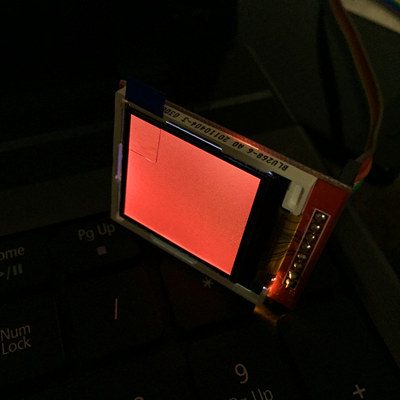### 原理说明

def write_command(cmd):
GPIO.output(cs, False)
GPIO.output(rs, False)
setByteData(cmd)
GPIO.output(cs, True)

def write_data(data):
GPIO.output(cs, False)
GPIO.output(rs, True)
setByteData(data)
GPIO.output(cs, True)

• 0x2A：指定绘图指针的列起始和结束地址（X坐标范围）
指令内容是4个字节的32位数据，从高位到低位分别是
起始X坐标高8位
起始X坐标低8位
结束X坐标高8位
结束X坐标低8位
• 0x2B：指定绘图指针的行起始和结束地址（Y坐标范围）
指令内容是4个字节的32位数据，从高位到低位分别是
起始Y坐标高8位
起始Y坐标低8位
结束Y坐标高8位
结束Y坐标低8位

write_command(0x2A) # Set Column Address
write_data(0x00)
write_data(0x00)
write_data(0x00)
write_data(0x7F)
write_data(0x00)
write_data(0x00)
write_data(0x00)
write_data(0x7F)
• 0x2C：在当前指针位置（X,Y坐标）绘制指定颜色的像素点
指令内容是2个字节的颜色数据。
每次执行完一个像素点的绘色以后，硬件会根据设定好的开始坐标和结束坐标自动移动绘图指针到下一个位置，遇到边界自动换行。
下面的代码的意义是连续绘制128 X 128个像素点，并且颜色为红色（#0xF800）。

write_command(0x2C)
show_single_color(0xf8,0x00) # 红色背景
...
...
def show_single_color(DH,DL):
for i in xrange(0,128):
for j in xrange(0,128):
write_data_16bit(DH,DL)

这就是本文示例代码显示红屏的原理。
其实只要学会了这3个命令的使用，基本上啥都能显示了，无非是效率问题。给大家留个作业，自己完成本文开头的最终效果彩带。
本节到此结束，下一节我们继续讨论。

### 关于颜色的补充说明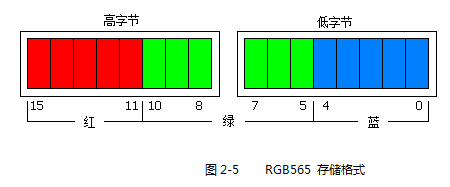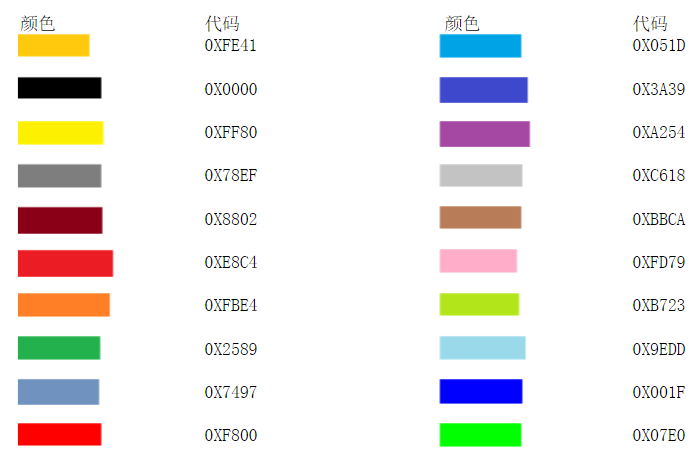• 4k Colors, RGB 4,4,4
3AH=“03h”

write_command(0x3a)
write_data(0x03)
• 65k Colors, RGB 5,6,5
3AH=“05h”（本文使用的格式）

write_command(0x3a)
write_data(0x05)
• 262k Colors, RGB 6,6,6
3AH=“06h”

write_command(0x3a)
write_data(0x06)